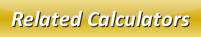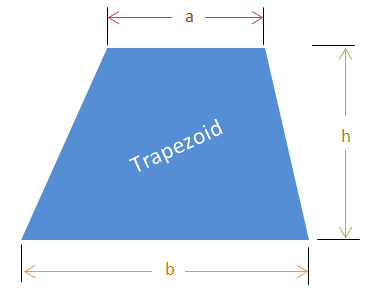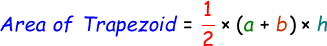# Area of a Trapezoid Calculator

A trapezoid also known as trapezium in some countries, is a special quadrilateral where one pair of sides are parallel. Below is an illustrations of trapezoid.We can calculate its area with the help of this below formula:where,
a = Upper horizontal length
b = Lower base horizontal length
h = Vertical height

In the below online area of a trapezium calculator, enter the given parameters and click calculate button to find the result.

 Upper Horizontal Length (a): Lower Base Horizontal Length (b): Vertical Height (h): Area of Trapezoid:

Latest Calculator Release

Average Acceleration Calculator

Average acceleration is the object's change in speed for a specific given time period. ...

Free Fall Calculator

When an object falls into the ground due to planet's own gravitational force is known a...

Torque Calculator

Torque is nothing but a rotational force. In other words, the amount of force applied t...

Average Force Calculator

Average force can be explained as the amount of force exerted by the body moving at giv...

Angular Displacement Calculator

Angular displacement is the angle at which an object moves on a circular path. It is de...